Download Presentation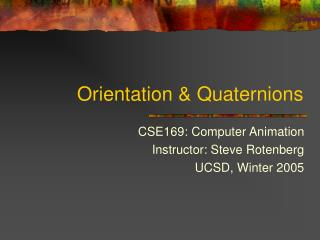Orientation & Quaternions

# Orientation & Quaternions - PowerPoint PPT Presentation

Orientation & Quaternions. CSE169: Computer Animation Instructor: Steve Rotenberg UCSD, Winter 2005. Orientation. Orientation. We will define ‘orientation’ to mean an object’s instantaneous rotational configuration Think of it as the rotational equivalent of position.I am the owner, or an agent authorized to act on behalf of the owner, of the copyrighted work described.
Download Presentation## Orientation & Quaternions

An Image/Link below is provided (as is) to download presentation

Download Policy: Content on the Website is provided to you AS IS for your information and personal use and may not be sold / licensed / shared on other websites without getting consent from its author.While downloading, if for some reason you are not able to download a presentation, the publisher may have deleted the file from their server.

- - - - - - - - - - - - - - - - - - - - - - - - - - E N D - - - - - - - - - - - - - - - - - - - - - - - - - -
Presentation Transcript### Orientation & Quaternions

CSE169: Computer Animation

Instructor: Steve Rotenberg

UCSD, Winter 2005### Orientation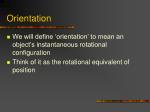Orientation
• We will define ‘orientation’ to mean an object’s instantaneous rotational configuration
• Think of it as the rotational equivalent of position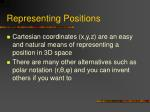Representing Positions
• Cartesian coordinates (x,y,z) are an easy and natural means of representing a position in 3D space
• There are many other alternatives such as polar notation (r,θ,φ) and you can invent others if you want to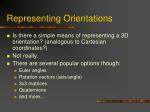Representing Orientations
• Is there a simple means of representing a 3D orientation? (analogous to Cartesian coordinates?)
• Not really.
• There are several popular options though:
• Euler angles
• Rotation vectors (axis/angle)
• 3x3 matrices
• Quaternions
• and more…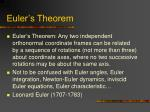Euler’s Theorem
• Euler’s Theorem: Any two independent orthonormal coordinate frames can be related by a sequence of rotations (not more than three) about coordinate axes, where no two successive rotations may be about the same axis.
• Not to be confused with Euler angles, Euler integration, Newton-Euler dynamics, inviscid Euler equations, Euler characteristic…
• Leonard Euler (1707-1783)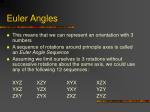Euler Angles
• This means that we can represent an orientation with 3 numbers
• A sequence of rotations around principle axes is called an Euler Angle Sequence
• Assuming we limit ourselves to 3 rotations without successive rotations about the same axis, we could use any of the following 12 sequences:

XYZ XZY XYX XZX

YXZ YZX YXY YZY

ZXY ZYX ZXZ ZYZ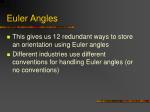Euler Angles
• This gives us 12 redundant ways to store an orientation using Euler angles
• Different industries use different conventions for handling Euler angles (or no conventions)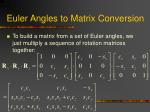Euler Angles to Matrix Conversion
• To build a matrix from a set of Euler angles, we just multiply a sequence of rotation matrices together: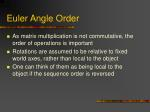Euler Angle Order
• As matrix multiplication is not commutative, the order of operations is important
• Rotations are assumed to be relative to fixed world axes, rather than local to the object
• One can think of them as being local to the object if the sequence order is reversedUsing Euler Angles
• To use Euler angles, one must choose which of the 12 representations they want
• There may be some practical differences between them and the best sequence may depend on what exactly you are trying to accomplishVehicle Orientation
• Generally, for vehicles, it is most convenient to rotate in roll (z), pitch (x), and then yaw (y)
• In situations where there

is a definite ground plane,

Euler angles can actually

be an intuitive

representation

front of vehicleGimbal Lock
• One potential problem that they can suffer from is ‘gimbal lock’
• This results when two axes effectively line up, resulting in a temporary loss of a degree of freedom
• This is related to the singularities in longitude that you get at the north and south polesInterpolating Euler Angles
• One can simply interpolate between the three values independently
• This will result in the interpolation following a different path depending on which of the 12 schemes you choose
• This may or may not be a problem, depending on your situation
• Interpolating near the ‘poles’ can be problematic
• Note: when interpolating angles, remember to check for crossing the +180/-180 degree boundariesEuler Angles
• Euler angles are used in a lot of applications, but they tend to require some rather arbitrary decisions
• They also do not interpolate in a consistent way (but this isn’t always bad)
• They can suffer from Gimbal lock and related problems
• There is no simple way to concatenate rotations
• Conversion to/from a matrix requires several trigonometry operations
• They are compact (requiring only 3 numbers)Rotation Vectors and Axis/Angle
• Euler’s Theorem also shows that any two orientations can be related by a single rotation about some axis (not necessarily a principle axis)
• This means that we can represent an arbitrary orientation as a rotation about some unit axis by some angle (4 numbers) (Axis/Angle form)
• Alternately, we can scale the axis by the angle and compact it down to a single 3D vector (Rotation vector)Axis/Angle to Matrix
• To generate a matrix as a rotation θ around an arbitrary unit axis a:Rotation Vectors
• To convert a scaled rotation vector to a matrix, one would have to extract the magnitude out of it and then rotate around the normalized axis
• Normally, rotation vector format is more useful for representing angular velocities and angular accelerations, rather than angular position (orientation)Axis/Angle Representation
• Storing an orientation as an axis and an angle uses 4 numbers, but Euler’s theorem says that we only need 3 numbers to represent an orientation
• Mathematically, this means that we are using 4 degrees of freedom to represent a 3 degrees of freedom value
• This implies that there is possibly extra or redundant information in the axis/angle format
• The redundancy manifests itself in the magnitude of the axis vector. The magnitude carries no information, and so it is redundant. To remove the redundancy, we choose to normalize the axis, thus constraining the extra degree of freedomMatrix Representation
• We can use a 3x3 matrix to represent an orientation as well
• This means we now have 9 numbers instead of 3, and therefore, we have 6 extra degrees of freedom
• NOTE: We don’t use 4x4 matrices here, as those are mainly useful because they give us the ability to combine translations. We will not be concerned with translation today, so we will just think of 3x3 matrices.Matrix Representation
• Those extra 6 DOFs manifest themselves as 3 scales (x, y, and z) and 3 shears (xy, xz, and yz)
• If we assume the matrix represents a rigid transform (orthonormal), then we can constrain the extra 6 DOFsMatrix Representation
• Matrices are usually the most computationally efficient way to apply rotations to geometric data, and so most orientation representations ultimately need to be converted into a matrix in order to do anything useful (transform verts…)
• Why then, shouldn’t we just always use matrices?
• Numerical issues
• Storage issues
• User interaction issues
• Interpolation issues### QuaternionsQuaternions
• Quaternions are an interesting mathematical concept with a deep relationship with the foundations of algebra and number theory
• Invented by W.R.Hamilton in 1843
• In practice, they are most useful to us as a means of representing orientations
• A quaternion has 4 componentsQuaternions (Imaginary Space)
• Quaternions are actually an extension to complex numbers
• Of the 4 components, one is a ‘real’ scalar number, and the other 3 form a vector in imaginary ijk space!Quaternions (Scalar/Vector)
• Sometimes, they are written as the combination of a scalar value s and a vector value v

whereUnit Quaternions
• For convenience, we will use only unit length quaternions, as they will be sufficient for our purposes and make things a little easier
• These correspond to the set of vectors that form the ‘surface’ of a 4D hypersphere of radius 1
• The ‘surface’ is actually a 3D volume in 4D space, but it can sometimes be visualized as an extension to the concept of a 2D surface on a 3D sphereQuaternions as Rotations
• A quaternion can represent a rotation by an angle θ around a unit axis a:
• If a is unit length, then q will be alsoQuaternion to Matrix
• To convert a quaternion to a rotation matrix:Matrix to Quaternion
• Matrix to quaternion is not too bad, I just don’t have room for it here
• It involves a few ‘if’ statements, a square root, three divisions, and some other stuff
• See Sam Buss’s book (p.305) for the algorithmSpheres
• Think of a person standing on the surface of a big sphere (like a planet)
• From the person’s point of view, they can move in along two orthogonal axes (front/back) and (left/right)
• There is no perception of any fixed poles or longitude/latitude, because no matter which direction they face, they always have two orthogonal ways to go
• From their point of view, they might as well be moving on a infinite 2D plane, however if they go too far in one direction, they will come back to where they started!Hyperspheres
• Now extend this concept to moving in the hypersphere of unit quaternions
• The person now has three orthogonal directions to go
• No matter how they are oriented in this space, they can always go some combination of forward/backward, left/right and up/down
• If they go too far in any one direction, they will come back to where they startedHyperspheres
• Now consider that a person’s location on this hypersphere represents an orientation
• Any incremental movement along one of the orthogonal axes in curved space corresponds to an incremental rotation along an axis in real space (distances along the hypersphere correspond to angles in 3D space)
• Moving in some arbitrary direction corresponds to rotating around some arbitrary axis
• If you move too far in one direction, you come back to where you started (corresponding to rotating 360 degrees around any one axis)Hyperspheres
• A distance of x along the surface of the hypersphere corresponds to a rotation of angle 2x radians
• This means that moving along a 90 degree arc on the hypersphere corresponds to rotating an object by 180 degrees
• Traveling 180 degrees corresponds to a 360 degree rotation, thus getting you back to where you started
• This implies that q and -q correspond to the same orientationHyperspheres
• Consider what would happen if this was not the case, and if 180 degrees along the hypersphere corresponded to a 180 degree rotation
• This would mean that there is exactly one orientation that is 180 opposite to a reference orientation
• In reality, there is a continuum of possible orientations that are 180 away from a reference
• They can be found on the equator relative to any point on the hypersphereHyperspheres
• Also consider what happens if you rotate a book 180 around x, then 180 around y, and then 180 around z
• You end up back where you started
• This corresponds to traveling along a triangle on the hypersphere where each edge is a 90 degree arc, orthogonal to each other edgeQuaternion Dot Products
• The dot product of two quaternions works in the same way as the dot product of two vectors:
• The angle between two quaternions in 4D space is half the angle one would need to rotate from one orientation to the other in 3D spaceQuaternion Multiplication
• We can perform multiplication on quaternions if we expand them into their complex number form
• If q represents a rotation and q’ represents a rotation, then qq’ represents q rotated by q’
• This follows very similar rules as matrix multiplication (I.e., non-commutative)Quaternion Multiplication
• Note that two unit quaternions multiplied together will result in another unit quaternion
• This corresponds to the same property of complex numbers
• Remember that multiplication by complex numbers can be thought of as a rotation in the complex plane
• Quaternions extend the planar rotations of complex numbers to 3D rotations in spaceQuaternion Joints
• One can create a skeleton using quaternion joints
• One possibility is to simply allow a quaternion joint type and provide a local matrix function that takes a quaternion
• Another possibility is to also compute the world matrices as quaternion multiplications. This involves a little less math than matrices, but may not prove to be significantly faster. Also, one would still have to handle the joint offsets with matrix mathQuaternions in the Pose Vector
• Using quaternions in the skeleton adds some complications, as they can’t simply be treated as 4 independent DOFs through the rig
• The reason is that the 4 numbers are not independent, and so an animation system would have to handle them specifically as a quaternion
• To deal with this, one might have to extend the concept of the pose vector as containing an array of scalars and an array of quaternions
• When higher level animation code blends and manipulates poses, it will have to treat quaternions specially### Quaternion InterpolationLinear Interpolation
• If we want to do a linear interpolation between two points a and b in normal space

Lerp(t,a,b) = (1-t)a + (t)b

where t ranges from 0 to 1

• Note that the Lerp operation can be thought of as a weighted average (convex)
• We could also write it in it’s additive blend form:

Lerp(t,a,b) = a + t(b-a)Spherical Linear Interpolation
• If we want to interpolate between two points on a sphere (or hypersphere), we don’t just want to Lerp between them
• Instead, we will travel across the surface of the sphere by following a ‘great arc’Spherical Linear Interpolation
• We define the spherical linear interpolation of two unit vectors in N dimensional space as:Quaternion Interpolation
• Remember that there are two redundant vectors in quaternion space for every unique orientation in 3D space
• What is the difference between:

Slerp(t,a,b) and Slerp(t,-a,b) ?

• One of these will travel less than 90 degrees while the other will travel more than 90 degrees across the sphere
• This corresponds to rotating the ‘short way’ or the ‘long way’
• Usually, we want to take the short way, so we negate one of them if their dot product is < 0Bezier Curves in 2D & 3D Space
• Bezier curves can be thought of as a higher order extension of linear interpolation

p1

p1

p2

p3

p1

p0

p0

p0

p2de Castlejau Algorithm

p1

• Find the point x on the curve as a function of parameter t:

p0

p2

p3Bezier Curves in Quaternion Space
• We can construct Bezier curves on the 4D hypersphere by following the exact same procedure using Slerp instead of Lerp
• It’s a good idea to flip (negate) the input quaternions as necessary in order to make it go the ‘short way’
• There are other, more sophisticated curve interpolation algorithms that can be applied to a hypersphere
• Interpolate several key poses
• Additional control over angular velocity, angular acceleration, smoothness…Quaternion Summary
• Quaternions are 4D vectors that can represent 3D rigid body orientations
• We choose to force them to be unit length
• Key animation functions:
• Quaternion-to-matrix / matrix-to-quaternion
• Quaternion multiplication: faster than matrix multiplication
• Slerp: interpolate between arbitrary orientations
• Spherical curves: de Castlejau algorithm for cubic Bezier curves on the hypersphereQuaternion References
• “Animating Rotation with Quaternion Curves”, Ken Shoemake, SIGGRAPH 1985
• “Quaternions and Rotation Sequences”, Kuipers